References:

## Approaches for Computing Derivatives¶

• Symbolic differentiation: automatic manipulation of mathematical expressions to get derivatives
• Takes a math expression and returns a math expression: $f(x) = x^2 \rightarrow \frac{df(x)}{dx} = 2x$
• Used in Mathematica, Maple, Sympy, etc.
• Numeric differentiation: Approximating derivatives by finite differences: $$\frac{\partial}{\partial x_i} f(x_1, \dots, x_N) = \lim_{h \to 0} \frac{f(x_1, \dots, x_i + h, \dots, x_N) - f(x_1, \dots, x_i - h, \dots, x_N)}{2h}$$
• Automatic differentiation: Takes code that computes a function and returns code that computes the derivative of that function.
• Reverse Mode AD: A method to get exact derivatives efficiently, by storing information as you go forward that you can reuse as you go backwards
• "The goal isn't to obtain closed-form solutions, but to be able to wirte a program that efficiently computes the derivatives." - Lecture 6 Slides (Backpropagation)

## Reverse Mode Automatic Differentiation¶

In machine learning, we have functions that have large fan-in, e.g. a neural net can have millions of parameters, that all squeeze down to one scalar that tells you how well it predicts something. cats.

### General Idea for Implementation¶

• Create a "tape" data structure that tracks the operations performed in computing a function
• Add themselves to the tape when called
• Compute gradients with respect to their local inputs
• Forward pass computes the function, and adds operations to the tape
• Reverse pass accumulates the local gradients using the chain rule
• This is efficient for graphs with large fan-in, like most loss functions in ML

• Autograd is a Python package for automatic differentiation.
          pip install autograd
• There are a lot of great examples provided with the source code

• Autograd can automatically differentiate native Python and Numpy code.
• It can handle a large subset of Python's features, including loops, conditional statements (if/else), recursion and closures
• It can also compute higher-order derivatives
• It uses reverse-mode differentiation (a.k.a. backpropagation) so it can efficiently take gradients of scalar-valued functions with respect to array-valued arguments.

## Autograd vs Tensorflow, Theano, etc.¶

Many deep learning packages implement automatic differentiation using small domain-specific languages within Python:

- Theano
- Caffe
- Vanilla Torch (as compared to Autograd for Torch)
- Tensorflow


Most of these alternatives require you to explicitly construct a computation graph; Autograd constructs a computation graph implicitly, by tracking the sequence of operations that have been performed during the execution of a program.

There are a couple projects to look into if you are interested in GPU support for Autograd:

In :
import autograd.numpy as np # Import thinly-wrapped numpy

In :
# Define a function like normal, using Python and Numpy
def tanh(x):
y = np.exp(-x)
return (1.0 - y) / (1.0 + y)

# Create a *function* that computes the gradient of tanh

# Evaluate the gradient at x = 1.0

# Compare to numeric gradient computed using finite differences
print((tanh(1.0001) - tanh(0.9999)) / 0.0002)

0.393223866483
0.393223866365


In this example, we will see how a complicated computation can be written as a composition of simpler functions, and how this provides a scalable strategy for computing gradients using the chain rule.

Say we want to write a function to compute the gradient of the sigmoid function: $$\sigma(x) = \frac{1}{1 + e^{-x}}$$ We can write $\sigma(x)$ as a composition of several elementary functions, as $\sigma(x) = s(c(b(a(x))))$, where:

$$a(x) = -x$$$$b(a) = e^a$$$$c(b) = 1 + b$$$$s(c) = \frac{1}{c}$$

Here, we have "staged" the computation such that it contains several intermediate variables, each of which are basic expressions for which we can easily compute the local gradients.

The computation graph for this expression is shown in the figure below.The input to this function is $x$, and the output is represented by node $s$. We wish compute the gradient of $s$ with respect to $x$, $\frac{\partial s}{\partial x}$. In order to make use of our intermediate computations, we can use the chain rule as follows: $$\frac{\partial s}{\partial x} = \frac{\partial s}{\partial c} \frac{\partial c}{\partial b} \frac{\partial b}{\partial a} \frac{\partial a}{\partial x}$$

In :
def grad_sigmoid_manual(x):
"""Implements the gradient of the logistic sigmoid function
$\sigma(x) = 1 / (1 + e^{-x})$ using staged computation
"""
# Forward pass, keeping track of intermediate values for use in the
# backward pass
a = -x         # -x in denominator
b = np.exp(a)  # e^{-x} in denominator
c = 1 + b      # 1 + e^{-x} in denominator
s = 1.0 / c    # Final result, 1.0 / (1 + e^{-x})

# Backward pass
dsdc = (-1.0 / (c**2))
dsdb = dsdc * 1
dsda = dsdb * np.exp(a)
dsdx = dsda * (-1)

return dsdx

def sigmoid(x):
y = 1.0 / (1.0 + np.exp(-x))
return y

# Compare the results of manual and automatic gradient functions:

0.104993585404
0.104993585404


## Gradients of Data Structures: flatten and unflatten¶

Autograd allows you to compute gradients for many different data structures. Autograd provides a lot of flexibility in the types of data structures you can use to store the parameters of your model. This flexibility is achieved through the flatten function, which converts any nested combination of lists, tuples, arrays, or dicts into a 1-dimensional Numpy array.

The idea is that we know how to compute gradients of vectors, and we can convert many data structures into vectors (i.e. "flatten" the data structures).

In :
import autograd.numpy as np


You can flatten a list of tuples:

In :
params = [(1.0, 1.0), (2.0,2.0), (3.0,3.0,3.0)]
flat_params, unflatten_func = flatten(params)
print('Flattened: {}'.format(flat_params))
print('Unflattened: {}'.format(unflatten_func(flat_params)))

Flattened: [ 1.  1.  2.  2.  3.  3.  3.]
Unflattened: [(array(1.0), array(1.0)), (array(2.0), array(2.0)), (array(3.0), array(3.0), array(3.0))]


A list of matrices (of different sizes):

In :
params = [npr.randn(3,3), npr.randn(4,4), npr.randn(3,3)]
flat_params, unflatten_func = flatten(params)
print('Flattened: {}'.format(flat_params))
print('Unflattened: {}'.format(unflatten_func(flat_params)))

Flattened: [-0.86718318 -0.39787176 -0.14008288  1.17582056 -2.20750251 -0.90372716
0.28830369 -0.71615753  0.44306775 -0.23534406  0.77017371 -0.82302491
-0.38458041  0.4164451  -2.0029848   0.19021276 -1.15892003 -0.56156495
0.52243588 -0.09529192  0.59047335  0.33227221 -1.15584222  0.90891582
1.79415157 -0.76722565 -0.41225893  0.61663727  0.8957365  -0.42721445
-1.41281425  0.32935995 -1.43127652  1.0173327 ]
Unflattened: [array([[-0.86718318, -0.39787176, -0.14008288],
[ 1.17582056, -2.20750251, -0.90372716],
[ 0.28830369, -0.71615753,  0.44306775]]), array([[-0.23534406,  0.77017371, -0.82302491, -0.38458041],
[ 0.4164451 , -2.0029848 ,  0.19021276, -1.15892003],
[-0.56156495,  0.52243588, -0.09529192,  0.59047335],
[ 0.33227221, -1.15584222,  0.90891582,  1.79415157]]), array([[-0.76722565, -0.41225893,  0.61663727],
[ 0.8957365 , -0.42721445, -1.41281425],
[ 0.32935995, -1.43127652,  1.0173327 ]])]


A dictionary:

In :
params = { 'weights': [1.0,2.0,3.0,4.0], 'biases': [1.0,2.0] }
flat_params, unflatten_func = flatten(params)
print('Flattened: {}'.format(flat_params))
print('Unflattened: {}'.format(unflatten_func(flat_params)))

Flattened: [ 1.  2.  1.  2.  3.  4.]
Unflattened: {'weights': [array(1.0), array(2.0), array(3.0), array(4.0)], 'biases': [array(1.0), array(2.0)]}


Or even a dictionary of dictionaries (etc.):

In :
params = { 'layer1': { 'weights': [1.0,2.0,3.0,4.0], 'biases': [1.0,2.0]}, 'layer2': { 'weights': [5.0,6.0,7.0,8.0], 'biases': [6.0,7.0]} }
flat_params, unflatten_func = flatten(params)
print('Flattened: {}'.format(flat_params))
print('Unflattened: {}'.format(unflatten_func(flat_params)))

Flattened: [ 1.  2.  1.  2.  3.  4.  6.  7.  5.  6.  7.  8.]
Unflattened: {'layer1': {'weights': [array(1.0), array(2.0), array(3.0), array(4.0)], 'biases': [array(1.0), array(2.0)]}, 'layer2': {'weights': [array(5.0), array(6.0), array(7.0), array(8.0)], 'biases': [array(6.0), array(7.0)]}}


There are several functions that compute gradients, which have different signatures

• grad(fun, argnum=0)
• Returns a function which computes the gradient of fun with respect to positional argument number argnum. The returned function takes the same arguments as fun, but returns the gradient instead. The function fun should be scalar-valued. The gradient has the same type as the argument.
• grad_named(fun, argname)
• Takes gradients with respect to a named argument.
• multigrad(fun, argnums=)
• Takes gradients wrt multiple arguments simultaneously.
• multigrad_dict(fun)
• Takes gradients with respect to all arguments simultaneously, and returns a dict mapping argname to gradval

One thing you can do is define custom gradients for your own functions. There are several reasons you might want to do this, including:

1. Speed: You may know a faster way to compute the gradient for a specific function.
2. Numerical Stability
3. When your code depends on external library calls

The @primitive decorator wraps a function so that its gradient can be specified manually and its invocation can be recorded.

In :
import autograd.numpy as np

# @primitive tells autograd not to look inside this function, but instead
# to treat it as a black box, whose gradient might be specified later.
@primitive
def logsumexp(x):
"""Numerically stable log(sum(exp(x)))"""
max_x = np.max(x)
return max_x + np.log(np.sum(np.exp(x - max_x)))

# Next, we write a function that specifies the gradient with a closure.
# If you want to be able to take higher-order derivatives, then all the
# code inside this function must be itself differentiable by autograd.
return np.full(x.shape, g) * np.exp(x - np.full(x.shape, ans))


In :
# Now we can use logsumexp() inside a larger function that we want to differentiate.
def example_func(y):
z = y**2
lse = logsumexp(z)
return np.sum(lse)

# Check the gradients numerically, just to be safe.
# Fails if a mismatch occurs

Gradient:  [ 0.01964729 -0.01052768  0.08082344  0.02737456  0.07575397 -0.01597028
3.0434424   0.00794668  0.00345275 -0.05588763]


# Examples¶

The next three sections of the notebook show examples of using Autograd in the context of three problems:

1. 1-D linear regression, where we try to fit a model to a function $y = wx + b$
2. Linear regression using a polynomial feature map, to fit a function of the form $y = w_0 + w_1 x + w_2 x^2 + \dots + w_M x^M$
3. Nonlinear regression using a neural network

## Linear Regression¶

### Review¶

We are given a set of data points $\{ (x_1, t_1), (x_2, t_2), \dots, (x_N, t_N) \}$, where each point $(x_i, t_i)$ consists of an input value $x_i$ and a target value $t_i$.

The model we use is: $$y_i = wx_i + b$$

We want each predicted value $y_i$ to be close to the ground truth value $t_i$. In linear regression, we use squared error to quantify the disagreement between $y_i$ and $t_i$. The loss function for a single example is: $$\mathcal{L}(y_i,t_i) = \frac{1}{2} (y_i - t_i)^2$$

The cost function is the loss averaged over all the training examples: $$\mathcal{E}(w,b) = \frac{1}{N} \sum_{i=1}^N \mathcal{L}(y_i, t_i) = \frac{1}{N} \sum_{i=1}^N \frac{1}{2} \left(wx_i + b - t_i \right)^2$$

In :
import autograd.numpy as np # Import wrapped NumPy from Autograd

import matplotlib.pyplot as plt # For plotting

%matplotlib inline


## Generate Synthetic Data¶

We generate a synthetic dataset $\{ (x_i, t_i) \}$ by first taking the $x_i$ to be linearly spaced in the range $[0, 10]$ and generating the corresponding value of $t_i$ using the following equation (where $w = 4$ and $b=10$): $$t_i = 4 x_i + 10 + \epsilon$$

Here, $\epsilon \sim \mathcal{N}(0, 2)$ (that is, $\epsilon$ is drawn from a Gaussian distribution with mean 0 and variance 2). This introduces some random fluctuation in the data, to mimic real data that has an underlying regularity, but for which individual observations are corrupted by random noise.

In :
# In our synthetic data, we have w = 4 and b = 10
N = 100 # Number of training data points
x = np.linspace(0, 10, N)
t = 4 * x + 10 + npr.normal(0, 2, x.shape)
plt.plot(x, t, 'r.')

Out:
[<matplotlib.lines.Line2D at 0x10ec4eeb8>]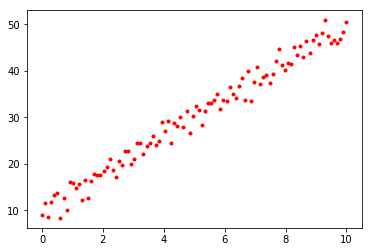In :
# Initialize random parameters
w = npr.normal(0, 1)
b = npr.normal(0, 1)
params = { 'w': w, 'b': b } # One option: aggregate parameters in a dictionary

def cost(params):
y = params['w'] * x + params['b']
return (1 / N) * np.sum(0.5 * np.square(y - t))

num_epochs = 1000  # Number of epochs of training
alpha = 0.01       # Learning rate

for i in range(num_epochs):
# Evaluate the gradient of the current parameters stored in params

# Update parameters w and b
params['w'] = params['w'] - alpha * cost_params['w']
params['b'] = params['b'] - alpha * cost_params['b']

print(params)

{'w': 4.1287788378129608, 'b': 9.1779559871908081}

In :
# Plot the training data again, together with the line defined by y = wx + b
# where w and b are our final learned parameters
plt.plot(x, t, 'r.')
plt.plot([0, 10], [params['b'], params['w'] * 10 + params['b']], 'b-')

Out:
[<matplotlib.lines.Line2D at 0x10f09a9b0>]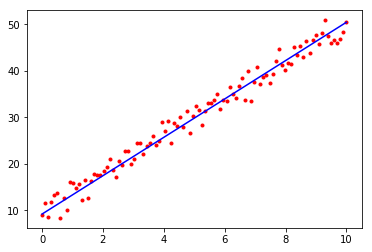## Linear Regression with a Feature Mapping¶

In this example we will fit a polynomial using linear regression with a polynomial feature mapping. The target function is:

$$t = x^4 - 10 x^2 + 10 x + \epsilon$$

where $\epsilon \sim \mathcal{N}(0, 4)$.

This is an example of a generalized linear model, in which we perform a fixed nonlinear transformation of the inputs $\mathbf{x} = (x_1, x_2, \dots, x_D)$, and the model is still linear in the parameters. We can define a set of feature mappings (also called feature functions or basis functions) $\phi$ to implement the fixed transformations.

In this case, we have $x \in \mathbb{R}$, and we define the feature mapping: $$\mathbf{\phi}(x) = \begin{pmatrix}\phi_1(x) \\ \phi_2(x) \\ \phi_3(x) \\ \phi_4(x) \end{pmatrix} = \begin{pmatrix}1\\x\\x^2\\x^3\end{pmatrix}$$

In :
# Generate synthetic data
N = 100 # Number of data points
x = np.linspace(-3, 3, N) # Generate N values linearly-spaced between -3 and 3
t = x ** 4 - 10 * x ** 2 + 10 * x + npr.normal(0, 4, x.shape) # Generate corresponding targets
plt.plot(x, t, 'r.') # Plot data points

Out:
[<matplotlib.lines.Line2D at 0x10f2b90f0>]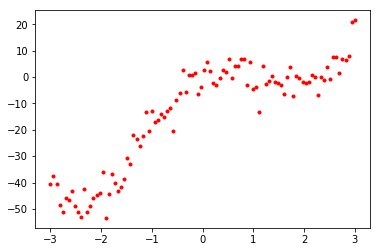In :
M = 4 # Degree of polynomial to fit to the data (this is a hyperparameter)
feature_matrix = np.array([[item ** i for i in range(M+1)] for item in x]) # Construct a feature matrix
W = npr.randn(feature_matrix.shape[-1])

def cost(W):
y = np.dot(feature_matrix, W)
return (1.0 / N) * np.sum(0.5 * np.square(y - t))

num_epochs = 10000
learning_rate = 0.001

for i in range(num_epochs):
W = W - learning_rate * cost_grad(W)

# Print the final learned parameters.
print(W)

[ -0.68443928  10.33612329  -9.48556594  -0.04644112   0.93365519]

In :
# Plot the original training data again, together with the polynomial we fit
plt.plot(x, t, 'r.')
plt.plot(x, np.dot(feature_matrix, W), 'b-')

Out:
[<matplotlib.lines.Line2D at 0x10f2f4048>]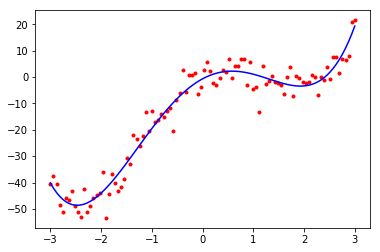## Neural Net Regression¶

In this example we will implement a (nonlinear) regression model using a neural network. To implement and train a neural net using Autograd, you only have to define the forward pass of the network and the loss function you wish to use; you do not need to implement the backward pass of the network. When you take the gradient of the loss function using grad, Autograd automatically computes computes the backward pass. It essentially executes the backpropagation algorithm implicitly.In :
import matplotlib.pyplot as plt

from autograd.misc import flatten #, flatten_func

%matplotlib inline


def sgd(grad, init_params, callback=None, num_iters=200, step_size=0.1, mass=0.9):
grad() must have signature grad(x, i), where i is the iteration number."""

velocity = np.zeros(len(x))
for i in range(num_iters):
if callback:
callback(unflatten(x), i, unflatten(g))
velocity = mass * velocity - (1.0 - mass) * g
x = x + step_size * velocity
return unflatten(x)

The next example shows how to use the sgd function.

In :
# Generate synthetic data
x = np.linspace(-5, 5, 1000)
t = x ** 3 - 20 * x + 10 + npr.normal(0, 4, x.shape)
plt.plot(x, t, 'r.')

Out:
[<matplotlib.lines.Line2D at 0x10f3c5dd8>]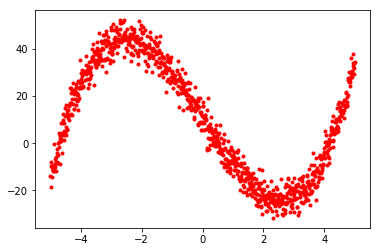In :
inputs = x.reshape(x.shape[-1],1)
W1 = npr.randn(1,4)
b1 = npr.randn(4)
W2 = npr.randn(4,4)
b2 = npr.randn(4)
W3 = npr.randn(4,1)
b3 = npr.randn(1)

params = { 'W1': W1, 'b1': b1, 'W2': W2, 'b2': b2, 'W3': W3, 'b3': b3 }

def relu(x):
return np.maximum(0, x)

nonlinearity = np.tanh
#nonlinearity = relu

def predict(params, inputs):
h1 = nonlinearity(np.dot(inputs, params['W1']) + params['b1'])
h2 = nonlinearity(np.dot(h1, params['W2']) + params['b2'])
output = np.dot(h2, params['W3']) + params['b3']
return output

def loss(params, i):
output = predict(params, inputs)
return (1.0 / inputs.shape) * np.sum(0.5 * np.square(output.reshape(output.shape) - t))

print(loss(params, 0))

optimized_params = sgd(grad(loss), params, step_size=0.01, num_iters=5000)
print(optimized_params)
print(loss(optimized_params, 0))

final_y = predict(optimized_params, inputs)
plt.plot(x, t, 'r.')
plt.plot(x, final_y, 'b-')

361.238545336
{'W1': array([[-1.04822356, -0.50365952, -0.99694494,  0.98227972]]), 'b1': array([ 4.78361845, -1.08061677,  1.27453929,  4.81991562]), 'W2': array([[-4.17283061, -3.25142667, -1.75741222, -3.65814981],
[ 2.03801363,  1.04339347, -2.17499704, -2.95023117],
[ 1.95205777,  0.87350044, -1.61815863, -2.90276696],
[ 0.28546842,  3.68840033,  4.15099628,  3.37168634]]), 'b2': array([ 3.12534049,  0.31222713,  1.16031296,  0.6667576 ]), 'W3': array([[ 13.56277971],
[ 18.00861121],
[ 11.14465696],
[ -5.91832481]]), 'b3': array([-1.30607817])}
7.99260111674

Out:
[<matplotlib.lines.Line2D at 0x10f2f9da0>]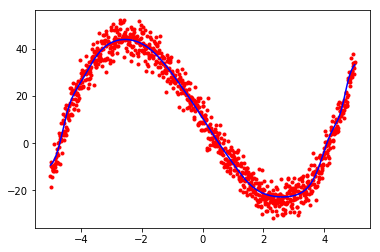In :
plt.plot(x, final_y, 'b-')

Out:
[<matplotlib.lines.Line2D at 0x10f587eb8>]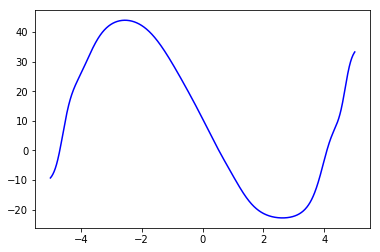In :
# A plot of the result of this model using ReLU activations
plt.plot(x, final_y, 'b-')

Out:
[<matplotlib.lines.Line2D at 0x10f6a5278>]In [ ]:


In [ ]: